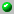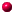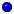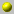Frank Sottile

The homepage can be accessed here.

Frank Sottile

Frank Sottile
Assistant Professor of Mathematics, University of Massachusetts, Amherst.
MSRI Program on Topological Aspects of Real Algebraic Varieties.
Anouncement.   Apply now for General Members, Postdocts, and Research Professorships.
Department of Mathematics and Statistics
University of Massachusetts
Amherst, MA   01003
Office: 1230 Lederle Graduate Research Tower
Telephone: 413-545-6010
FAX: 413-545-1801
Email: sottile@math.umass.edu

the following papers are available on our server= dvi format= ps format= tex format= pdf formata_geometric_approach_to_the_combinatorics_of_schubert_polynomialsa_monoid_for_the_universal_k_bruhat_ordera_pieri_type_formula_for_isotropic_flag_manifoldsenumerative_geometry_for_real_varietiesenumerative_geometry_for_the_real_grassmannian_of_lines_in_projective_spacehopf_algebras_and_edge_labeled_posetsidentities_of_structure_constants_for_schubert_polynomials_and_orders_of_snnumerical_schubert_calculuspieris_formula_via_explicit_rational_equivalencepieris_rule_for_flag_manifolds_and_schubert_polynomialspieri_type_formulas_for_maximal_isotropic_grassmannians_via_triple_intersectionsreal_enumerative_geometry_and_effective_algebraic_equivalence
schubert_polynomials_the_bruhat_order_and_the_geometry_of_flag_manifoldsschubert_polynomials_the_bruhat_order_and_the_geometry_of_flag_manifoldsshifted_quasi_symmetric_functions_and_the_hopf_algebra_of_peak_functionsskew_schubert_functions_and_the_pieri_formula_for_flag_manifoldsskew_schubert_polynomialstableau_switching_algorithms_and_applications

Go back to the directory of pre and reprints.
Or search the database by keywords
Or look for the newest entires in the database